## Using Right Triangles to Evaluate Trigonometric Functions

In earlier sections, we used a unit circle to define the trigonometric functions. In this section, we will extend those definitions so that we can apply them to right triangles. The value of the sine or cosine function of $t$ is its value at $t$ radians. First, we need to create our right triangle. Figure 1 shows a point on a unit circle of radius 1. If we drop a vertical line segment from the point $\left(x,y\right)\\$ to the x-axis, we have a right triangle whose vertical side has length $y$ and whose horizontal side has length $x$. We can use this right triangle to redefine sine, cosine, and the other trigonometric functions as ratios of the sides of a right triangle.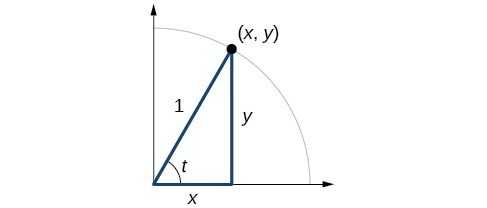Figure 1

We know

$\cos \text{ }t=\frac{x}{1}=x\\$

Likewise, we know

$\sin \text{ }t=\frac{y}{1}=y\\$

These ratios still apply to the sides of a right triangle when no unit circle is involved and when the triangle is not in standard position and is not being graphed using $\left(x,y\right)\\$ coordinates. To be able to use these ratios freely, we will give the sides more general names: Instead of $x$, we will call the side between the given angle and the right angle the adjacent side to angle $t$. (Adjacent means “next to.”) Instead of $y$, we will call the side most distant from the given angle the opposite side from angle $\text{}t$. And instead of $1$, we will call the side of a right triangle opposite the right angle the hypotenuse. These sides are labeled in Figure 2.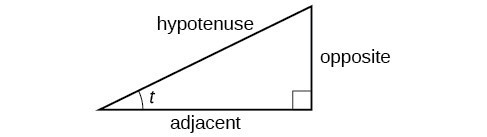Figure 2. The sides of a right triangle in relation to angle $t$.

## Understanding Right Triangle Relationships

Given a right triangle with an acute angle of $t$,

$\begin{array}{l}\sin \left(t\right)=\frac{\text{opposite}}{\text{hypotenuse}}\hfill \\ \cos \left(t\right)=\frac{\text{adjacent}}{\text{hypotenuse}}\hfill \\ \tan \left(t\right)=\frac{\text{opposite}}{\text{adjacent}}\hfill \end{array}\\$

A common mnemonic for remembering these relationships is SohCahToa, formed from the first letters of “Sine is opposite over hypotenuse, Cosine is adjacent over hypotenuse, Tangent is opposite over adjacent.”

### How To: Given the side lengths of a right triangle and one of the acute angles, find the sine, cosine, and tangent of that angle.

1. Find the sine as the ratio of the opposite side to the hypotenuse.
2. Find the cosine as the ratio of the adjacent side to the hypotenuse.
3. Find the tangent is the ratio of the opposite side to the adjacent side.

### Example 1: Evaluating a Trigonometric Function of a Right Triangle

Given the triangle shown in Figure 3, find the value of $\cos \alpha \\$.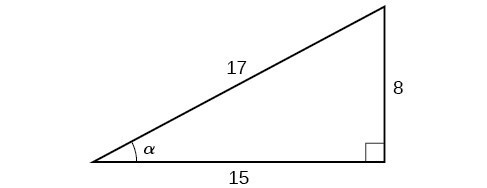Figure 3

### Solution

The side adjacent to the angle is 15, and the hypotenuse of the triangle is 17, so:

$\begin{array}{l}\cos \left(\alpha \right)=\frac{\text{adjacent}}{\text{hypotenuse}}\hfill \\ =\frac{15}{17}\hfill \end{array}\\$

### Try It 1

Given the triangle shown in Figure 4, find the value of $\text{sin}t$.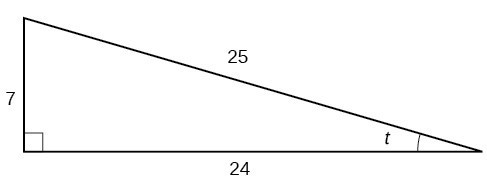Figure 4

Solution

## Relating Angles and Their Functions

When working with right triangles, the same rules apply regardless of the orientation of the triangle. In fact, we can evaluate the six trigonometric functions of either of the two acute angles in the triangle in Figure 5. The side opposite one acute angle is the side adjacent to the other acute angle, and vice versa.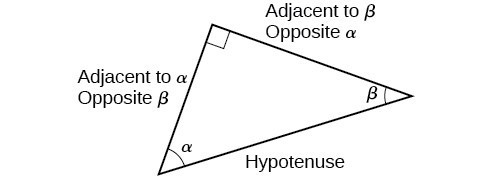Figure 5. The side adjacent to one angle is opposite the other.

We will be asked to find all six trigonometric functions for a given angle in a triangle. Our strategy is to find the sine, cosine, and tangent of the angles first. Then, we can find the other trigonometric functions easily because we know that the reciprocal of sine is cosecant, the reciprocal of cosine is secant, and the reciprocal of tangent is cotangent.

### How To: Given the side lengths of a right triangle, evaluate the six trigonometric functions of one of the acute angles.

1. If needed, draw the right triangle and label the angle provided.
2. Identify the angle, the adjacent side, the side opposite the angle, and the hypotenuse of the right triangle.
3. Find the required function:
• sine as the ratio of the opposite side to the hypotenuse
• cosine as the ratio of the adjacent side to the hypotenuse
• tangent as the ratio of the opposite side to the adjacent side
• secant as the ratio of the hypotenuse to the adjacent side
• cosecant as the ratio of the hypotenuse to the opposite side
• cotangent as the ratio of the adjacent side to the opposite side

### Example 2: Evaluating Trigonometric Functions of Angles Not in Standard Position

Using the triangle shown in Figure 6, evaluate $\sin \alpha \\$, $\cos \alpha \\$, $\tan \alpha \\$, $\sec \alpha\\$, $\csc \alpha$, and $\cot \alpha\\$.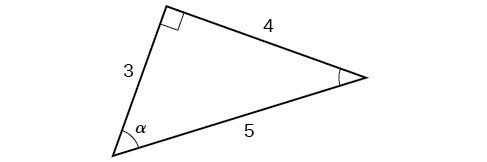Figure 6

### Solution

$\begin{array}{l}\sin \alpha =\frac{\text{opposite }\alpha }{\text{hypotenuse}}=\frac{4}{5}\hfill \\ \cos \alpha =\frac{\text{adjacent to }\alpha }{\text{hypotenuse}}=\frac{3}{5}\hfill \\ \tan \alpha =\frac{\text{opposite }\alpha }{\text{adjacent to }\alpha }=\frac{4}{3}\hfill \\ \sec \alpha =\frac{\text{hypotenuse}}{\text{adjacent to }\alpha }=\frac{5}{3}\hfill \\ \csc \alpha =\frac{\text{hypotenuse}}{\text{opposite }\alpha }=\frac{5}{4}\hfill \\ \cot \alpha =\frac{\text{adjacent to }\alpha }{\text{opposite }\alpha }=\frac{3}{4}\hfill \end{array}\\$

### Try It 2

Using the triangle shown in Figure 7, evaluate $\sin \text{ }t\\$, $\cos \text{ }t\\$, $\tan \text{ }t\\$, $\sec \text{ }t\\$, $\csc \text{ }t\\$, and $\cot \text{ }t\\$.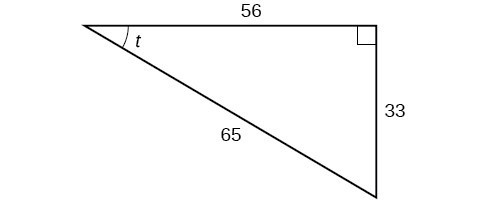Figure 7

Solution

## Finding Trigonometric Functions of Special Angles Using Side Lengths

We have already discussed the trigonometric functions as they relate to the special angles on the unit circle. Now, we can use those relationships to evaluate triangles that contain those special angles. We do this because when we evaluate the special angles in trigonometric functions, they have relatively friendly values, values that contain either no or just one square root in the ratio. Therefore, these are the angles often used in math and science problems. We will use multiples of $30^\circ$, $60^\circ$, and $45^\circ \\$, however, remember that when dealing with right triangles, we are limited to angles between $0^\circ \text{ and 90^\circ }\text{.}\\$

Suppose we have a $30^\circ ,60^\circ ,90^\circ \\$ triangle, which can also be described as a $\frac{\pi }{6},\text{ } \frac{\pi }{3},\frac{\pi }{2}\\$ triangle. The sides have lengths in the relation $s,\sqrt{3}s,2s\\$. The sides of a $45^\circ ,45^\circ ,90^\circ$ triangle, which can also be described as a $\frac{\pi }{4},\frac{\pi }{4},\frac{\pi }{2}\\$ triangle, have lengths in the relation $s,s,\sqrt{2}s\\$. These relations are shown in Figure 8.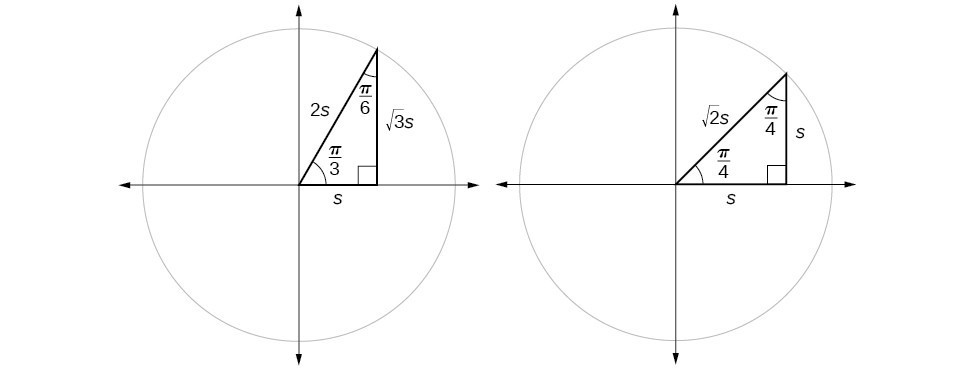Figure 8. Side lengths of special triangles

We can then use the ratios of the side lengths to evaluate trigonometric functions of special angles.

### How To: Given trigonometric functions of a special angle, evaluate using side lengths.

1. Use the side lengths shown in Figure 8 for the special angle you wish to evaluate.
2. Use the ratio of side lengths appropriate to the function you wish to evaluate.

### Example 3: Evaluating Trigonometric Functions of Special Angles Using Side Lengths

Find the exact value of the trigonometric functions of $\frac{\pi }{3}\\$, using side lengths.

### Solution

$\begin{array}{l}\sin \left(\frac{\pi }{3}\right)=\frac{\text{opp}}{\text{hyp}}=\frac{\sqrt{3}s}{2s}=\frac{\sqrt{3}}{2}\hfill \\ \cos \left(\frac{\pi }{3}\right)=\frac{\text{adj}}{\text{hyp}}=\frac{s}{2s}=\frac{1}{2}\hfill \\ \tan \left(\frac{\pi }{3}\right)=\frac{\text{opp}}{\text{adj}}=\frac{\sqrt{3}s}{s}=\sqrt{3}\hfill \\ \sec \left(\frac{\pi }{3}\right)=\frac{\text{hyp}}{\text{adj}}=\frac{2s}{s}=2\hfill \\ \csc \left(\frac{\pi }{3}\right)=\frac{\text{hyp}}{\text{opp}}=\frac{2s}{\sqrt{3}s}=\frac{2}{\sqrt{3}}=\frac{2\sqrt{3}}{3}\hfill \\ \cot \left(\frac{\pi }{3}\right)=\frac{\text{adj}}{\text{opp}}=\frac{s}{\sqrt{3}s}=\frac{1}{\sqrt{3}}=\frac{\sqrt{3}}{3}\hfill \end{array}\\$

### Try It 3

Find the exact value of the trigonometric functions of $\frac{\pi }{4}\\$, using side lengths.

Solution

## Using Equal Cofunction of Complements

If we look more closely at the relationship between the sine and cosine of the special angles relative to the unit circle, we will notice a pattern. In a right triangle with angles of $\frac{\pi }{6}$ and $\frac{\pi }{3}$, we see that the sine of $\frac{\pi }{3}$, namely $\frac{\sqrt{3}}{2}$, is also the cosine of $\frac{\pi }{6}$, while the sine of $\frac{\pi }{6}$, namely $\frac{1}{2}$, is also the cosine of $\frac{\pi }{3}$.

$\begin{array}{l}\begin{array}{l}\hfill \\ \sin \frac{\pi }{3}=\cos \frac{\pi }{6}=\frac{\sqrt{3}s}{2s}=\frac{\sqrt{3}}{2}\hfill \end{array}\hfill \\ \sin \frac{\pi }{6}=\cos \frac{\pi }{3}=\frac{s}{2s}=\frac{1}{2}\hfill \end{array}$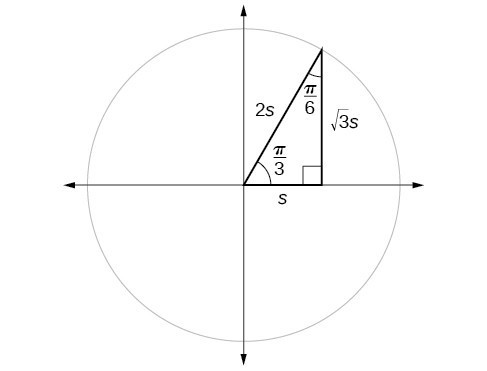Figure 9. The sine of $\frac{\pi }{3}$ equals the cosine of $\frac{\pi }{6}$ and vice versa.

This result should not be surprising because, as we see from Figure 9, the side opposite the angle of $\frac{\pi }{3}$ is also the side adjacent to $\frac{\pi }{6}$, so $\sin \left(\frac{\pi }{3}\right)$ and $\cos \left(\frac{\pi }{6}\right)$ are exactly the same ratio of the same two sides, $\sqrt{3}s$ and $2s$. Similarly, $\cos \left(\frac{\pi }{3}\right)$ and $\sin \left(\frac{\pi }{6}\right)$ are also the same ratio using the same two sides, $s$ and $2s$.

The interrelationship between the sines and cosines of $\frac{\pi }{6}$ and $\frac{\pi }{3}$ also holds for the two acute angles in any right triangle, since in every case, the ratio of the same two sides would constitute the sine of one angle and the cosine of the other. Since the three angles of a triangle add to $\pi$, and the right angle is $\frac{\pi }{2}$, the remaining two angles must also add up to $\frac{\pi }{2}$. That means that a right triangle can be formed with any two angles that add to $\frac{\pi }{2}$ —in other words, any two complementary angles. So we may state a cofunction identity: If any two angles are complementary, the sine of one is the cosine of the other, and vice versa. This identity is illustrated in Figure 10.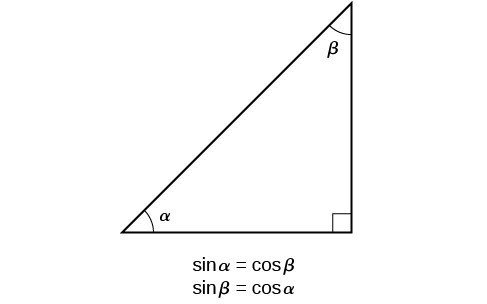Figure 10. Cofunction identity of sine and cosine of complementary angles

Using this identity, we can state without calculating, for instance, that the sine of $\frac{\pi }{12}$ equals the cosine of $\frac{5\pi }{12}$, and that the sine of $\frac{5\pi }{12}$ equals the cosine of $\frac{\pi }{12}$. We can also state that if, for a certain angle $t$, $\cos \text{ }t=\frac{5}{13}$, then $\sin \left(\frac{\pi }{2}-t\right)=\frac{5}{13}$ as well.

### A General Note: Cofunction Identities

The cofunction identities in radians are listed in the table below.

 $\cos t=\sin \left(\frac{\pi }{2}-t\right)$ $\sin t=\cos \left(\frac{\pi }{2}-t\right)$ $\tan t=\cot \left(\frac{\pi }{2}-t\right)$ $\cot t=\tan \left(\frac{\pi }{2}-t\right)$ $\sec t=\csc \left(\frac{\pi }{2}-t\right)$ $\csc t=\sec \left(\frac{\pi }{2}-t\right)$

### How To: Given the sine and cosine of an angle, find the sine or cosine of its complement.

1. To find the sine of the complementary angle, find the cosine of the original angle.
2. To find the cosine of the complementary angle, find the sine of the original angle.

### Example 4: Using Cofunction Identities

If $\sin t=\frac{5}{12}$, find $\left(\cos \frac{\pi }{2}-t\right)$.

### Solution

According to the cofunction identities for sine and cosine,

$\sin t=\cos \left(\frac{\pi }{2}-t\right)$.

So

$\cos \left(\frac{\pi }{2}-t\right)=\frac{5}{12}$.

### Try It 4

If $\csc \left(\frac{\pi }{6}\right)=2$, find $\sec \left(\frac{\pi }{3}\right)$.

Solution

## Using Trigonometric Functions

In previous examples, we evaluated the sine and cosine in triangles where we knew all three sides. But the real power of right-triangle trigonometry emerges when we look at triangles in which we know an angle but do not know all the sides.

### How To: Given a right triangle, the length of one side, and the measure of one acute angle, find the remaining sides.

1. For each side, select the trigonometric function that has the unknown side as either the numerator or the denominator. The known side will in turn be the denominator or the numerator.
2. Write an equation setting the function value of the known angle equal to the ratio of the corresponding sides.
3. Using the value of the trigonometric function and the known side length, solve for the missing side length.

### Example 5: Finding Missing Side Lengths Using Trigonometric Ratios

Find the unknown sides of the triangle in Figure 11.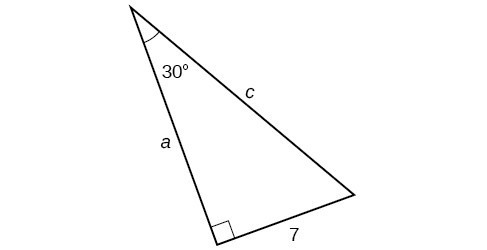Figure 11

### Solution

We know the angle and the opposite side, so we can use the tangent to find the adjacent side.

$\tan \left(30^\circ \right)=\frac{7}{a}$

We rearrange to solve for $a$.

$\begin{array}{l}a=\frac{7}{\tan \left(30^\circ \right)}\hfill \\ =12.1\hfill \end{array}$

We can use the sine to find the hypotenuse.

$\sin \left(30^\circ \right)=\frac{7}{c}$

Again, we rearrange to solve for $c$.

$\begin{array}{l}c=\frac{7}{\sin \left(30^\circ \right)}\hfill \\ =14\hfill \end{array}$

### Try It 5

A right triangle has one angle of $\frac{\pi }{3}$ and a hypotenuse of 20. Find the unknown sides and angle of the triangle.

Solution

Right-triangle trigonometry has many practical applications. For example, the ability to compute the lengths of sides of a triangle makes it possible to find the height of a tall object without climbing to the top or having to extend a tape measure along its height. We do so by measuring a distance from the base of the object to a point on the ground some distance away, where we can look up to the top of the tall object at an angle. The angle of elevation of an object above an observer relative to the observer is the angle between the horizontal and the line from the object to the observer’s eye. The right triangle this position creates has sides that represent the unknown height, the measured distance from the base, and the angled line of sight from the ground to the top of the object. Knowing the measured distance to the base of the object and the angle of the line of sight, we can use trigonometric functions to calculate the unknown height. Similarly, we can form a triangle from the top of a tall object by looking downward. The angle of depression of an object below an observer relative to the observer is the angle between the horizontal and the line from the object to the observer’s eye.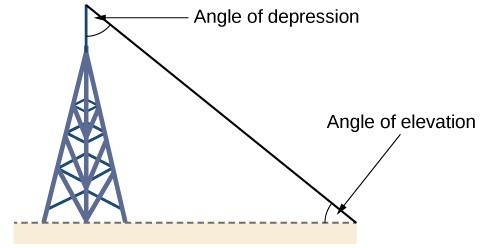Figure 12

### How To: Given a tall object, measure its height indirectly.

1. Make a sketch of the problem situation to keep track of known and unknown information.
2. Lay out a measured distance from the base of the object to a point where the top of the object is clearly visible.
3. At the other end of the measured distance, look up to the top of the object. Measure the angle the line of sight makes with the horizontal.
4. Write an equation relating the unknown height, the measured distance, and the tangent of the angle of the line of sight.
5. Solve the equation for the unknown height.

### Example 6: Measuring a Distance Indirectly

To find the height of a tree, a person walks to a point 30 feet from the base of the tree. She measures an angle of $57^\circ$ between a line of sight to the top of the tree and the ground, as shown in Figure 13. Find the height of the tree.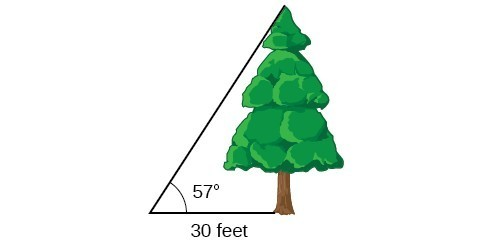Figure 13

### Solution

We know that the angle of elevation is $57^\circ$ and the adjacent side is 30 ft long. The opposite side is the unknown height.

The trigonometric function relating the side opposite to an angle and the side adjacent to the angle is the tangent. So we will state our information in terms of the tangent of $57^\circ$, letting $h$ be the unknown height.

$\begin{array}{ll}\tan \theta =\frac{\text{opposite}}{\text{adjacent}}\hfill & \hfill \\ \text{tan}\left(57^\circ \right)=\frac{h}{30}\hfill & \text{Solve for }h.\hfill \\ h=30\tan \left(57^\circ \right)\hfill & \text{Multiply}.\hfill \\ h\approx 46.2\hfill & \text{Use a calculator}.\hfill \end{array}$

The tree is approximately 46 feet tall.

### Try It 6

How long a ladder is needed to reach a windowsill 50 feet above the ground if the ladder rests against the building making an angle of $\frac{5\pi }{12}$ with the ground? Round to the nearest foot.

Solution

## Key Equations

 Cofunction Identities $\begin{array}{l}\begin{array}{l}\\ \cos t=\sin \left(\frac{\pi }{2}-t\right)\end{array}\hfill \\ \sin t=\cos \left(\frac{\pi }{2}-t\right)\hfill \\ \tan t=\cot \left(\frac{\pi }{2}-t\right)\hfill \\ \cot t=\tan \left(\frac{\pi }{2}-t\right)\hfill \\ \sec t=\csc \left(\frac{\pi }{2}-t\right)\hfill \\ \csc t=\sec \left(\frac{\pi }{2}-t\right)\hfill \end{array}$

## Key Concepts

• We can define trigonometric functions as ratios of the side lengths of a right triangle.
• The same side lengths can be used to evaluate the trigonometric functions of either acute angle in a right triangle.
• We can evaluate the trigonometric functions of special angles, knowing the side lengths of the triangles in which they occur.
• Any two complementary angles could be the two acute angles of a right triangle.
• If two angles are complementary, the cofunction identities state that the sine of one equals the cosine of the other and vice versa.
• We can use trigonometric functions of an angle to find unknown side lengths.
• Select the trigonometric function representing the ratio of the unknown side to the known side.
• Right-triangle trigonometry permits the measurement of inaccessible heights and distances.
• The unknown height or distance can be found by creating a right triangle in which the unknown height or distance is one of the sides, and another side and angle are known.

## Glossary

in a right triangle, the side between a given angle and the right angle
angle of depression
the angle between the horizontal and the line from the object to the observer’s eye, assuming the object is positioned lower than the observer
angle of elevation
the angle between the horizontal and the line from the object to the observer’s eye, assuming the object is positioned higher than the observer
opposite side
in a right triangle, the side most distant from a given angle
hypotenuse
the side of a right triangle opposite the right angle

## Section Exercises

1. For the given right triangle, label the adjacent side, opposite side, and hypotenuse for the indicated angle.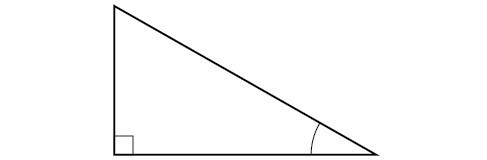2. When a right triangle with a hypotenuse of 1 is placed in the unit circle, which sides of the triangle correspond to the x– and y-coordinates?

3. The tangent of an angle compares which sides of the right triangle?

4. What is the relationship between the two acute angles in a right triangle?

5. Explain the cofunction identity.

For the following exercises, use cofunctions of complementary angles.

6. $\cos \left(\text{34^\circ }\right)=\sin \left(\text{__^\circ }\right)$

7. $\cos \left(\frac{\pi }{3}\right)=\sin \text{(___)}$

8. $\csc \left(\text{21^\circ }\right)=\sec \left(\text{___^\circ }\right)$

9. $\tan \left(\frac{\pi }{4}\right)=\cot \left(\text{__}\right)$

For the following exercises, find the lengths of the missing sides if side $a$ is opposite angle $A$, side $b$ is opposite angle $B$, and side $c$ is the hypotenuse.

10. $\cos B=\frac{4}{5},a=10$

11. $\sin B=\frac{1}{2}, a=20$

12. $\tan A=\frac{5}{12},b=6$

13. $\tan A=100,b=100$

14. $\sin B=\frac{1}{\sqrt{3}}, a=2$

15. $a=5,\measuredangle A={60}^{\circ }$

16. $c=12,\measuredangle A={45}^{\circ }$

For the following exercises, use Figure 14 to evaluate each trigonometric function of angle $A$.

17. $\sin A$

18. $\cos A$

19. $\tan A$

20. $\csc A$

21. $\sec A$

22. $\cot A$

For the following exercises, use Figure 15 to evaluate each trigonometric function of angle $A$.

23. $\sin A$

24. $\cos A$

25. $\tan A$

26. $\csc A$

27. $\sec A$

28. $\cot A$

For the following exercises, solve for the unknown sides of the given triangle.

29.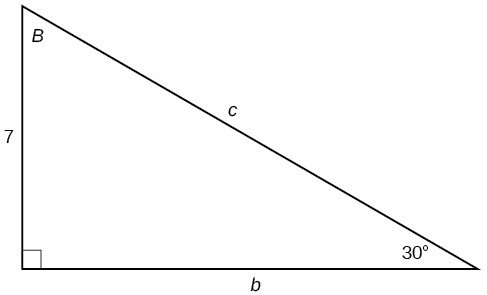30.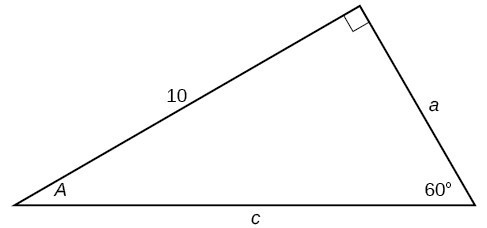31.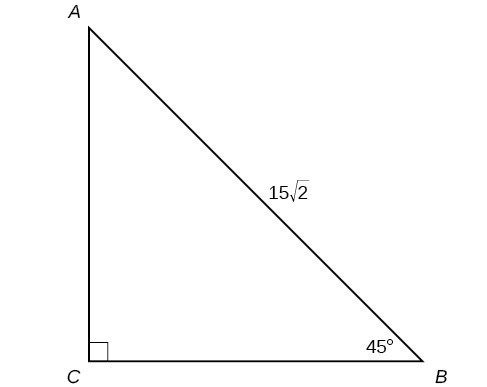For the following exercises, use a calculator to find the length of each side to four decimal places.

32.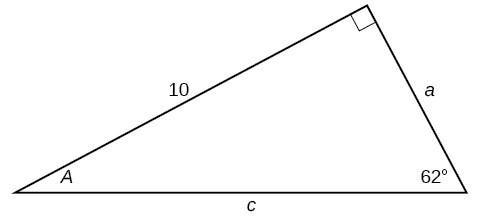33.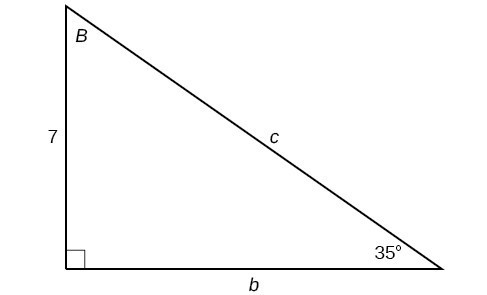34.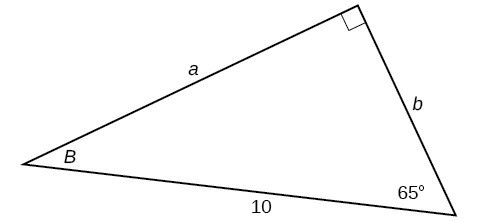35.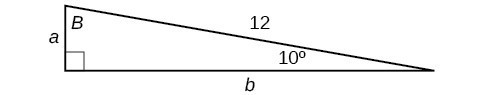36.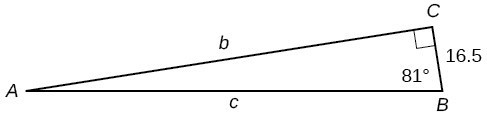37. $b=15,\measuredangle B={15}^{\circ }$

38. $c=200,\measuredangle B={5}^{\circ }$

39. $c=50,\measuredangle B={21}^{\circ }$

40. $a=30,\measuredangle A={27}^{\circ }$

41. $b=3.5,\measuredangle A={78}^{\circ }$

42. Find $x$.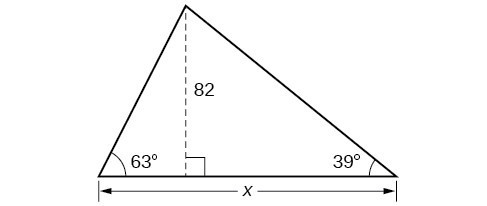43. Find $x$.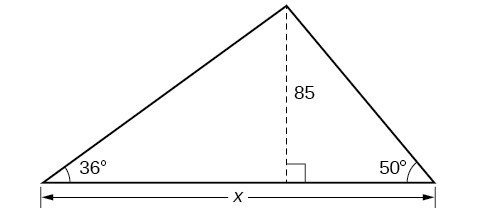44. Find $x$.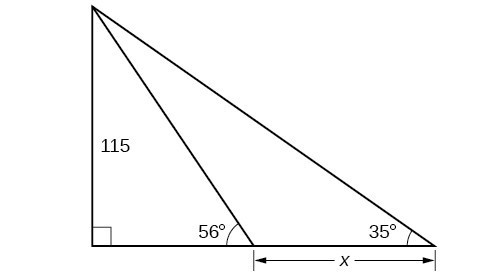45. Find $x$.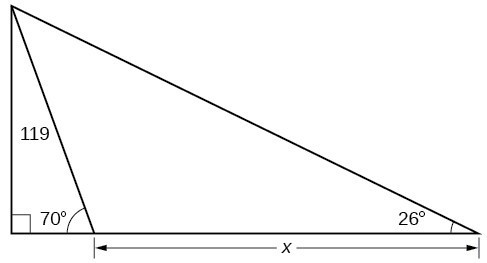46. A radio tower is located 400 feet from a building. From a window in the building, a person determines that the angle of elevation to the top of the tower is $36^\circ$, and that the angle of depression to the bottom of the tower is $23^\circ$. How tall is the tower?

47. A radio tower is located 325 feet from a building. From a window in the building, a person determines that the angle of elevation to the top of the tower is $43^\circ$, and that the angle of depression to the bottom of the tower is $31^\circ$. How tall is the tower?

48. A 200-foot tall monument is located in the distance. From a window in a building, a person determines that the angle of elevation to the top of the monument is $15^\circ$, and that the angle of depression to the bottom of the tower is $2^\circ$. How far is the person from the monument?

49. A 400-foot tall monument is located in the distance. From a window in a building, a person determines that the angle of elevation to the top of the monument is $18^\circ$, and that the angle of depression to the bottom of the tower is $3^\circ$. How far is the person from the monument?

50. There is an antenna on the top of a building. From a location 300 feet from the base of the building, the angle of elevation to the top of the building is measured to be $40^\circ$. From the same location, the angle of elevation to the top of the antenna is measured to be $43^\circ$. Find the height of the antenna.

51. There is lightning rod on the top of a building. From a location 500 feet from the base of the building, the angle of elevation to the top of the building is measured to be $36^\circ$. From the same location, the angle of elevation to the top of the lightning rod is measured to be $38^\circ$. Find the height of the lightning rod.

52. A 33-ft ladder leans against a building so that the angle between the ground and the ladder is $80^\circ$. How high does the ladder reach up the side of the building?

53. A 23-ft ladder leans against a building so that the angle between the ground and the ladder is $80^\circ$. How high does the ladder reach up the side of the building?

54. The angle of elevation to the top of a building in New York is found to be 9 degrees from the ground at a distance of 1 mile from the base of the building. Using this information, find the height of the building.

55. The angle of elevation to the top of a building in Seattle is found to be 2 degrees from the ground at a distance of 2 miles from the base of the building. Using this information, find the height of the building.

56. Assuming that a 370-foot tall giant redwood grows vertically, if I walk a certain distance from the tree and measure the angle of elevation to the top of the tree to be $60^\circ$, how far from the base of the tree am I?